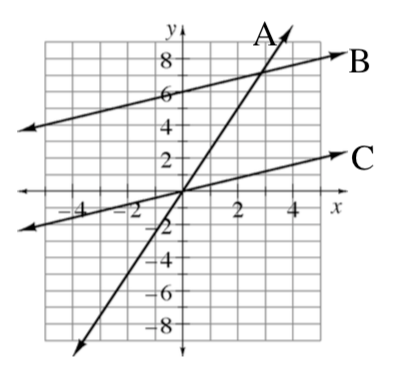### Home > CC3 > Chapter 7 > Lesson 7.2.2 > Problem7-49

7-49.

Compare the graphs of lines A, B, and C at right.

Which line increases most sharply?

Line A

1. What is the $y$-value of line A when $x=2$?

Locate line A and $x=2$ on the graph.

What is the $y$-coordinate of the point on the graph?

1. Compare the slopes of lines B and C.

The lines are parallel. Parallel lines have the same change in $y$ for the same change in $x$.